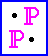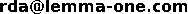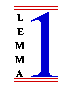# Supplementary ProofPower Examples

Contributing Documentation Download Examples Getting Home Mailing List Papers Patches Specifications
This page contains links to a selection of documents giving examples of specification and proof using ProofPower, supplementing the examples for ProofPower-HOL and ProofPower-Z supplied with the system itself.

The examples are supplied as PDF documents for you to read and as source tarballs so that you can experiment with them using ProofPower.

## ProofPower-HOL: FEF - a Security Application

The FEF project has a page all to itself including instructions for downloading the FEF source.

## ProofPower-HOL: Some Mathematical Case Studies

The tarball containing the source for these case studies is available for download here. To replay the specifications and proofs, we recommend you use the latest version of the case study and of ProofPOwer. The case studies comprise the following documents:

### wrk066.pdf - Basic Analysis

This ProofPower-HOL document contains definitions and proofs concerning some of the basics of analysis. The material covered includes: polynomial functions on the real numbers, limits of sequences, continuity of functions of real variables, differentiation, limits of function values, uniform convergence of limits of functions, series and power series and their use in defining the exponential, logarithm and sine and cosine functions and Archimedes' constant, π other circular and hyperbolic trigonometric functions and a selection of their inverse functions; definition and basic properties of the Henstock-Kurzweil gauge integral including the fundamental theorem of the calculus; calculation of the areas of some simple plane sets; Buffon's needle problem.

### wrk067.pdf - Some Topology

This ProofPower-HOL document contains definitions and proofs concerning some basics of abstract topology, metric space theory and algebraic topology (more precisely, elementary homotopy theory). The material currently covers the standard basic facts about compactness, connectedness etc. together with enough metric space theory to get started on the topology of the real line and the plane and develop some homotopy theory as far as the theorems that say the fundamental groupoid satisfies the groupoid laws, that the fundamental group is a group and that covering projections are fibrations.

### wrk068.pdf - Some Group Theory

This ProofPower-HOL document contains definitions and proofs concerning the elements of group theory. What is currently covered is what might be covered in the first chapter of a typical introductory text on the subject: in preparation for the introduction of quotient groups, we begin with a purely set-theoretical study of equivalance relations and the quotient of a set with respect to an equivalence relation. This is followed by the definitions of the concepts of group, homomorphism between groups, subgroup, normal subgroup, kernel of a homomorphism, congruence modulo a subgroup, coset of a subgroup, and quotient group. Theorems proved included the three isomorphism theorems, the Cayley representation theorem and Lagrange's theorem. Several examples of groups are exhibited and used to show that the various abstract notions lead to the expected theorems: e.g., that the exponential function is an isomorphism between the additive group of real numbers and the multiplicative group of positive real numbers.

### wrk072.pdf The Complex Numbers

This ProofPower-HOL document contains definitions and proofs concerning elementary algebra in the complex numbers. This includes the definitions of the field operations, of complex conjugation and of the embeddings of the reals and naturals; the proof that the complex numbers are indeed a field; proofs that complex conjugation and the embeddings of the other number systems are homomorphisms; proof of de Moivre's theorem. We define polynomial functions and prove various theorems about them. We prove that the exponential mapping from the real line to the unit circle in the complex plane is a covering projection. We define the degree of a self-mapping of a circle and use it to prove the fundamental theorem of algebra.

### wrk073.pdf Some Finite and Infinite Combinatorics

This document presents some definitions and theorems from elementary finite and infinite combinatorics. The definitions include a "fold" operator for finite sets and the operation that sums a real-valued function on a finite indexed set. The theorems include: more facts about finiteness and the size of finite sets; algebraic properties of indexed sums; induction over finitely-supported functions; the inclusion/exclusion principle; the binomial coefficients and their basic properties, including the formula for the number of combinations and the binomial theorem. The theory is applied to give proofs of Bertrand's ballot theorem and the two-square theorem. The main theorems provided in infinite combinatorics are the infinite versions of the pigeon-hole principle and of Ramsey's theorem.

### wrk074.pdf Some Number Theory

This ProofPower-HOL document presents some definitions and theorems from number theory in ProofPower-HOL. The topics currently covered include: divisors and greatest common divisors; Euclid's algorithm; the infinitude of the primes; the fundamental theorem of arithmetic; divergence of the series of prime reciprocals; Wilson's theorem.

### wrk076.pdf The Geometric Algebra

A construction of the Geometric Algebra.

## ProofPower-Z: Theory of Refinement

The source for these case studies is available for download here. To replay the specifications and proofs, we recommend you use the latest version of the case study and of ProofPOwer. The case studies comprise the following documents:

### wrk069.pdf - On Refinement Calculus and Partial Correctness

This note is intended as the first of a series concerned with a style of system specification in which the claims that can be verified have the form ``system p satisfies specification s under normal circumstances'', i.e., the notion of partial correctness. It presents a generic definition of (partial) refinement and develops the notations needed to state and prove that the resulting refinement ordering forms a complete lattice. The relationship of refinement to relational inclusion is investigated.

### wrk070.pdf - On Correctness of Imperative Programs - Precondition Calculation

This note is the second in a planned series concerned with specification via pre- and post-conditions in a partial correctness setting. It applies the general notion of specification and refinement given in the first note in the series to give a notion of correctness for a simple imperative programming language, where programs and program fragments may be equipped with Floyd-Hoare style specification annotations. The note presents a simple formal model in ProofPower-Z of a refinement notation comprising a miniature, but complete, imperative programming language annotated with formal specifications; the semantics of that programming language and the notion of correctness relative to the specification annotations is defined. A semantic model of a verification condition generator (or pre-condition calculator) is given which can be proved to be sound with respect both to the programming language semantics and to the intensional semantics of the specification annotations.

### wrk075.pdf - A Traced Monoidal Category of Relations

This note is the third in a series concerned with specification via pre- and post-conditions. It sets up a generic framework for systems formed by wiring together primitive building blocks. Such systems will be modelled as relations using existentially quantified equations for the fixed points that correspond to feedback loops in the wiring diagram. The approach is based on the notion of traced monoidal categories. The category-phobic reader should not despair as the discussion here is self-contained, and the relatively simple ideas that fall under this fancy name do provide a very nice general algebraic way of thinking about diagrammatic models.

## ProofPower-Z: SMT-LIB in Z

The source for this example is available for download here. To replay the specifications and proofs, we recommend you use the latest version of the case study and of ProofPOwer.

### zsmtlib.pdf - SMT-LIB in Z

This document gives a model written in Z of each of the theories defined by the SMT-LIB standard. It also covers some of the extensions defined in the SMT-LIB logics including, in particular, all of the operations of the bit vector logic. The source document also includes a few proofs, e.g., to show that the ProofPower-Z definition of integer division agrees with the SMT-LIB definition.

## ProofPower-Z: Proof Examples

The source for these case studies is available for download here. To replay the specifications and proofs, we recommend you use the latest version of the case study and of ProofPOwer. The miscellany currently comprises just one document but some more should be coming soon.

### wrk071.pdf - The Mutilated Chessboard Theorem in Z

This note presents a statement and proof of the mutilated chessboard theorem in Z. The formulation is along the lines of the one proposed by McCarthy for a `heavy duty set theory'' theorem prover. The theorem and its proof are presented as a Z specification and a series of Z conjectures all of which have been mechanically verified using the ProofPower system. For the entertainment of the theorem-proving community, we conclude by proposing a rather harder challenge problem.

 Copyright © Lemma 1 Ltd. Created by  Rob ArthanLast updated: \$Date: 2017/10/13 12:12:59 \$